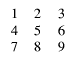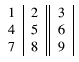2014. Nov. update

ps.revtex4-1 是 American Physical Society製作的documentclass(就是指一種文章的格式).

-- 寫Title

-- 寫authors

-- 寫authors' information (email, affiliation, homepage...)

-- 放圖片

-- 放表格

-- 放equation

-- 放footnote

-- 放reference

-- 放appendix

Latex是個很powerful的排版工具，但它需要花點時間學習，

revtex4-1的麻煩點：

1. Lyx 的documentclass選項中，沒有revtex4-1, 你要使用額外的Local layout.

這個revtex4-1.layout file 可以在這邊下載

下載後，把rextev4-1.layout放在跟你.tex檔相同的資料夾中，

開啟Lyx後選local layout，就可以用ctrl+R預覽成品了。

 Preamble % Fix a bug in REVTeX 4.1 \def\lovname{List of Videos} \@ifundefined{textcolor}{} {% \definecolor{BLACK}{gray}{0} \definecolor{WHITE}{gray}{1} \definecolor{RED}{rgb}{1,0,0} \definecolor{GREEN}{rgb}{0,1,0} \definecolor{BLUE}{rgb}{0,0,1} \definecolor{CYAN}{cmyk}{1,0,0,0} \definecolor{MAGENTA}{cmyk}{0,1,0,0} \definecolor{YELLOW}{cmyk}{0,0,1,0} }

ps. 這個revtex4-1.layout file中，我把原本內容的這段給刪掉了

因為，這段碼好像會一直讓編譯失敗

2. 作不出revtex4-1要求的作者格式3.作不出revtex4-1的表格Lyx可以export它專用的.lyx檔成 .tex檔，然後再到texmaker程式中作微調。本篇的內容：

-- 寫Title, authors ... 放圖片, 表格,...

http://en.wikibooks.org/wiki/LaTeX  (這邊的資料非常的豐富！也寫得很淺顯易懂）

ps...我是用virtualbox, host是windows7, guest是Ubuntu.

SECTION 1: Install Latex and revtex4-1

( 找不到synaptic的話，去ubuntu software中尋找加安裝就可以了)

open synapticSearch"revtex",

Install "texlive-publisher"packageSECTION 2: Compile the sample tex file.

/usr/share/doc/texlive-publishers-doc/latex/revtex/sample/apslatex apssamp.tex

bibtex apssamp

latex apssamp.tex

latex apssamp.tex

(ps. latex 需要complie數次，所以最後兩個指令不是我多寫的，而是需要的)

(當我剛接觸revtex4-1時，光研究要怎麼安裝我就搞了2-3天晚上....)evince appsamp.dvi &pdflatex apssamp.tex ; evince apssamp.pdfSECTION 3-1: brief introduction -- Preamble"%" 的符號代表註解

aps, 是指 american physics soceity的規定

prc: physics review C 期刊的格式

( prl: physic review letter 期刊的格式)

twocolumn: 兩行的模式; 用於最後出版的

preprint: 單行模式; 用於peer-reviewing 比較好檢查錯誤

APS 還有寫好給 .bib 檔案(reference file)的style,

\bibliographystyle{apsrev4-1}

SECTION 3-2: brief introduction -- Authors, and Authors' information

Lyx在這部份作不出revtex4-1的格式，建議使用texmaker.

以下是我的設定：下面是email, homepage等等：\author{A}

\author{B}

\affiliation{ XXX university}

\author{A}

\email[ current email]{  OOO@gmail.com}

% [ ] 之內是放description, {} 之類是放value, like email address.

% 在頁面最下之處就會顯示出 作者A的email

\author{B}

\altafiliation{ present: UK}

% 是 alt + affiliation的意思，比方說某個作者畢業了，去新的地方工作，

% 那這資訊就可以放上去。

\date{\today}

% 會顯示日期，一般都是預設用today, 系統會自動顯示時間。

SECTION 3-3: brief introduction -- abstract

\begin{abstract}

\end{abstract}ps. The \maketitle command generates a title on a separate title page - except in the article style, where the title normally goes at the top of the first page.

SECTION 3-4: brief introduction -- structure

\documentclass[]{revtex4-1}
\bibliographystyle{apsrev4-1}

\begin{document}

\author{xination}
\affiliation{ XXX university}
\date{\today}

\begin{abstract}
\end{abstract}
\pacs{}
\maketitle

\section{這是第一段}
\subsection{還可以在細分}
\subsection{還可以在細分}

\section{這是第二段}
\subsection{還可以在細分}
\subsection{還可以在細分}

\section{我是第三段}
\subsection{還可以在細分}
\subsection{還可以在細分}

\begin{thebibliography}
\end{thebibliography}

\end{document}

ex.

\begin{figure}
...一堆設定
\end{figure}

or

\begin{table}
...一堆設定
\end{table}

SECTION 3-5: brief introduction -- label

\label的功能我們常常看到唷。

Section I

This is a string.\label{just a test string}

OK show me \ref{just a test string}.

\label{fig: pie chart}

\label{fig: scatter chart}

\label{eg: Schrodinger equation}

my example:SECTION 3-6: brief introduction -- Citation and Reference

\begin{thebibliography}
\end{thebibliography}

\end{document}

\begin{thebibliography} {9}

\bibitem{自己填的keyword1} C. Thibault, R. Klapisch, C. Rigaud, A. M. Poskanzer, R. Prieels, L. Lessard, and W. Reisdorf, Phys. Rev. C {\bf 12}, 644 (1975).

\end{thebibliography}

ps. {9}不是很重要，它是控制width的

The XXX experiment discovered OOOO \cite{自己填的keyword1}.

example:\begin{thebibliography}

\end{thebibliography}

latex apssamp.tex

bibtex apssamp

latex apssamp.tex

latex apssamp.tex

不然是會有問號出現的唷

subsection A: using bibtex under Lyx

Lyx 下都是圖形界面的，按一按就可完成設定。Lyx 會詢問你 Databases and style for .bib file.Then 用Browse找出你自己存取的.bib file.APS 有提供他們style file for .bib file; apsrev4-1.bstSECTION 3-7: brief introduction -- insert picture

\begin{figure*}
\begin{center}

\includegraphics[scale=.5]{file_path}
\caption{}
\label{}

\end{center}
\end{figure*}

{figure*}是給two column格式時用的，

scale是指要將原圖縮放的比例。

then file_path就是 figures/abc.jpg1) 先按insert->float->figure.  這樣會出現 float的environment,

\begin{figure*}
\caption{}
\end{figure*}

2) 先將滑鼠點到caption之外的地方，用下面的圖例表示，\begin{figure*}
\includegraphics[scale=.5]{demo.png}
\caption{this is tile}
\end{figure*}

3) 最後在全選框框裡面的東西，按右鍵用paragraph settings讓東西都置中

\begin{figure*}
\begin{center}

\includegraphics[scale=.5]{demo.png}
\caption{this is tile}

\end{center}
\end{figure*}

SECTION 3-8: brief introduction -- table and footnote

table的設計比較複雜，若有合併儲存格、形狀特殊的表格，那又更加麻煩了。

& 為column separator

\\ 為  start new row

\hline  為horizontal line

ps. "\," 為小小的space，是為了fine tune用的。

ps. "~ "也是space，為了fine tune用的。

example1

\begin{tabular}{ l c r %靠齊; l代表left, c代表center, r代表right
1 & 2 & 3 \\
4 & 5 & 6 \\
7 & 8 & 9 \\
\end{tabular}note: tabular 是用來控制 element 靠左、置中、靠右的。

lcr 代表:

example2

\begin{tabular}{ l | c || r | } %加了 pipe line "|" 就可以有solid line.
1 & 2 & 3 \\
4 & 5 & 6 \\
7 & 8 & 9 \\
\end{tabular}example3

\begin{center}  % 把表格置中
\begin{table}
\begin{tabular}{c c c}
\hline
\hline
A & B & C\\
\hline
1 &~~~~2~~~~~& 3 \\
4 & 5 & 6 \\
7 & 8 & 9 \\
\hline
\hline
\end{tabular}  \label{tab:number}
\end{table}
\end{center}my example:note: ruledtabular 是用來加入 double line的，他會自動出現在表格的最上與下方。

ex.

term1a

term2a

term3b

term4b

term1\footnote{ wowowow}

term2\footmaker

term3\footnote{ hahahaha}

term4\footmaker

table 太寬，就得用跨欄的方式，要使用float。

\begin{table*}
\end{table*}SECTION 3-9: brief introduction -- equation

equation的說明在很多地方都有，這邊列出常見的math symbol.

Ax  in latex:  $A_{x}$

Ax in latex:   $A^{x}$

Greek letter:

in latex: $\alpha$

in latex: $\beta$

You can also try the Online LaTex editor: check it.\begin{eqnarray}\label{eq:strange equation}

G^{k}\centerdot F_{k}=\left\{ \underset{a\neq b}{\sum}\left(\begin{array}{c}
5\\
a
\end{array}\right)\centerdot\left(\begin{array}{c}
7\\
b
\end{array}\right)\right\} \centerdot A_{i}^{5}\centerdot\sqrt{\pi^{3/2}}\nonumber\\
\times \int\int y^{2}\sin\left(x\right)dxdy+\theta\centerdot\frac{\partial}{\partial x}T(x,y)
\end{eqnarray}

\usepackage{amsmath}
\usepackage{amssymb}

\begin{eqnarray}
\end{eqnarray}的環境下，是會幫equation給標號的。SECTION 4: 結語

Merry Christmas~~~

Appendix:

Lyx的教學影片

Lyx基礎的操作